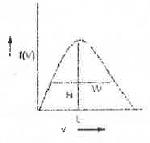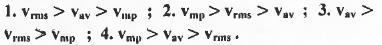Courses

# Past Year Questions: Theory of Gases IIT JAM Notes | EduRev

## IIT JAM : Past Year Questions: Theory of Gases IIT JAM Notes | EduRev

The document Past Year Questions: Theory of Gases IIT JAM Notes | EduRev is a part of the IIT JAM Course Physical Chemistry.
All you need of IIT JAM at this link: IIT JAM

Try yourself:The no. of normal modes of vibration of naphthalene is



Try yourself:From the kinetic theory of gases, the ratio of most probable speed (Cmp) to root mean square speed (Crms) is



Try yourself:The relationship between van der Waals 'b' co-efficient of N2 and O2 is

1. b(N2) = b(O2) = 0 ;

2. b(N2) = b(O2) ≠ 0 ;

3. b(N2) > b(O2) ;

4. b(N2) < b(O2)



Try yourself:According to the equipartition principle, the predicted high temperature limiting value of the molar heat capacity at constant volume for C2H2 is



Try yourself:Among (l)Ar, (2)NH4CI, (3)HF and (4)HCl ,the strength of interatomic/intermolecular forces follows the order:



Try yourself:According to the equipartition principle of energy, the molar heat capacity at constant volume for CO2(g), SO2(g) and H2O (g) follows the trend :

1. CO2 = SO2 = H2O ;

2. CO2 > SO2 = H2O ;

3. H2P > SP2 = CP2 ;

4. CO2 = SO2 > H2O



Try yourself:The average speed of H2, N2 and O2 gas molecules is in the order

1. H2 > N2 > O2 ;

2. O2 > N2 > H2 ;

3. H2 > O2 > N2 ;

4. N2 > O2 > H2.



Try yourself:Based on the principle of equipartition of energy, the molar heat capacity of CO2 at constant volume Cv,m is



Try yourself:The molar internal energy of a gas at temperature T is Um(T). The molar internal energy at T = 0 is Um(0). The correct expression that relates these two with appropriate contributions is

1. Um(T) = Um(0) + 3RT | Linear molecule ; translation only]

2. Um(T) = Um(0) + 5/2RT | Linear molecule ; translation and rotation only|

3. Um(T) = Um(0) + 3/2RT | Non-linear molecule ; translation and rotation only|

4. Um(T) = Um(0) + RT | Non-linear molecule ; translation only|.



Try yourself:Consider two identical containers, one with 1 mole of H2 and the other with 1 mole of He. If the root-mean-square ( RMS ) velocities of two gases are the same , then the ratio of the temperature T(H2)/T(He) is



Try yourself:For an ideal gas, the plot that is NONLINEAR is

1. PV vs. T ;

2. PV vs. P, at constant T ;

3. P vs. V, at constant T ;

4. In P vs. In V, at constant T.



Try yourself:Given that the most probable speed of oxygen gas is 1000 ms-1, the average speed under the same conditions is



Try yourself:The Maxwell-Boitzmann distribution for molecule speeds is shown in the following figure.In the figure, H is the height of the peak, L is the location of the maximum and W is the width at half height. As the temperature is decreased

1. H increases, L decreases and W increases ;

2. M Increases, L decreases and W decreases ;

3. H decreases, L Increases and W increases ;

4. H decreases, L decreases and W decreases.



Try yourself:The molar heat capacity at constant volume of a colourless gas is found to be 25 J/mol/K. at room temperature. The gas must be



Try yourself:For the distribution of molecular velocities of gases, identify the correct order from the following (where vmp, , vav and vrms are the most probable velocity , average velocity and root mean square velocity respectively):Offer running on EduRev: Apply code STAYHOME200 to get INR 200 off on our premium plan EduRev Infinity!

## Physical Chemistry

84 videos|106 docs|31 tests

,

,

,

,

,

,

,

,

,

,

,

,

,

,

,

,

,

,

,

,

,

;